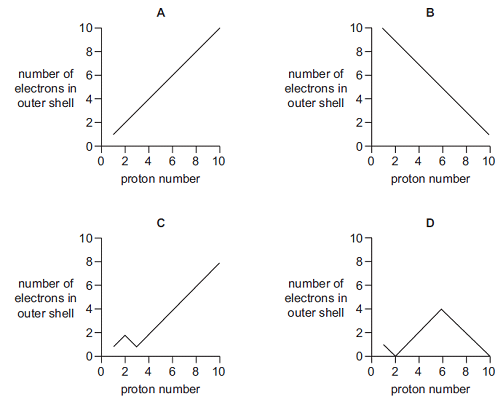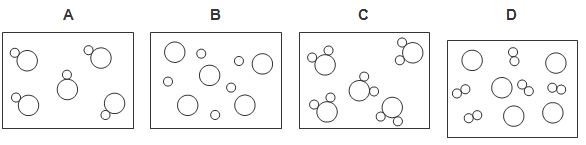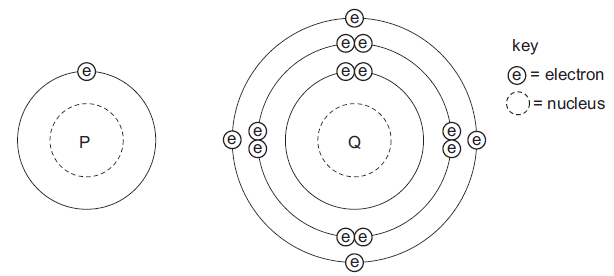1.Why is the first ionisation energy of phosphorus greater than the first ionisation energy of silicon?

2. Equations involving four enthalpy changes are shown.

Na(g) → Na+(g) + e     ΔH = W

Na(g) → Na2+(g) + 2e   ΔH = X

Na(s) → Na(g)           ΔH = Y

Na(s) → Na2+(g) + 2e    ΔH = Z

What is the second ionisation energy of sodium?

3.Alloys are usually harder than the metals from which they are made.Which difference between the metals explains the greater hardness of alloys?

4.Which graph shows the number of electrons in the outer shell of an atom, plotted against the proton (atomic) number for the first ten elements in the Periodic Table?5. Element X is in group 2, and element Y in group 7, of the periodic table.  Which ions will be present in the compound formed when X and Y react together?

6.Which of the following properties of the halogens increase from F to I?

2. Melting point

3.  Electronegativity

7.Which pair would react together most vigorously?

8.Which factor helps to explain why the first ionisation energies of the Group I elements decrease from lithium to sodium to potassium to rubidium?

9.In the diagrams, circles of different sizes represent atoms of different elements. Which diagram can represent hydrogen chloride gas?10. The diagram shows the electronic structures of atoms P and Q.P and Q combine to form a molecule.What is the formula of this molecule?

Question 1 of 10